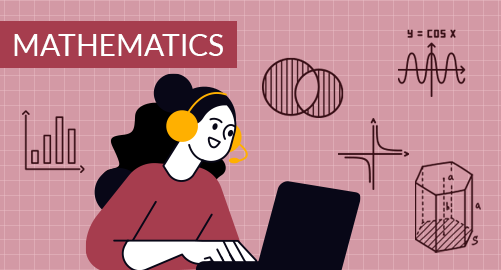#### through a demo today# Year 8 Mathematics

Mathematics
Year 8

The Year 8 Mathematics curriculum equips students with a variety of skills that are essential for mathematical development and applying mathematical concepts to real-life situations. It covers topics from numbers, fractions, decimals, percentages, financial math, ratios and rates, measurements, Pythagoras theorem, algebra, equations, and inequalities, straight line graphs geometry, transformation and congruence, data collection, representation and analysis, sets and Venn diagrams and probability.

## What you'll learn

• 1

Numbers system

• 2

Fractions, decimals, percentages, and financial mathematics

• 3

Ratio and rates

• 4

Measurements and Pythagoras theorem

• 5

Algebra

• 6

Equations and inequalities

• 7

Straight line graphs

• 8

Geometry

• 9

Transformation and congruence

• 10

Data collection, representation, and analysis

• 11

Sets and Venn diagrams

• 12

Probability

## Skills you'll learnNumeracy skills - Review of real numbers, finding square and cube roots, usage of prime factorisation, LCM, andHCF.Arithmetic operations - Basic arithmetic operations on fractions, decimals, percentages, conversion of fractions, and decimals into percentages and vice versa. Financial knowledge - understanding basic financial concepts, calculating, and applying GST, simple interest, profit and loss.Comparison skills - Learning ratios and rates enhance your ability to understand, compare, and manipulate quantities, helping to solve complex problems that involve real-life situations.Measurement skills - Measuring length, perimeter, and area of figures. Calculating surface area, volume and capacity of 3D figures. Understand and apply Pythagoras' theorem to solve real-life problems.Algebraic thinking - Understanding algebraic expressions and equations. Calculating unknown values, abstract thinking by working with symbols and variables, recognising and understanding patterns, developing visualisation skills through graphs, systematic problem-solvingAnalytical thinking - Understanding equality and inequality of relations gives problem-solving skills and enhances your ability to represent and solve real-world situations mathematically, providing a crucial foundation for advanced algebraic concepts and applications in various fields.Interpretational thinking - Straight line graphs develop skills in understanding and interpreting graphical representations of linear relationships, aiding in data analysis and making predictions based on trends.Geometrical thinking - Cultivates spatial reasoning and visualisation abilities, crucial for tasks like design, architecture, and engineering. It also develops logical thinking and problem-solving skills through the application of geometric principles and theorems.Visualisation and logical thinking - Strengthen your ability to analyse and manipulate objects in various orientations. It also sharpens problem-solving skills by applying geometric transformations and congruence principles.Data analysis and interpretation - Understanding the data and drawing conclusions, dot plots, column graphs, line graphs, travel graphs, sector graphs, bar graphs, frequency tables, frequency histograms, frequency polygons graphs, and charts. Calculate and measure central tendency (mean, median, and mode).Logical thinking - Categorising the elements in a set, drawing the Venn diagram based on given data, visualizing the Venn diagrams, manipulating the sets through union and intersection, and interpreting Venn diagrams in real-life situations.Critical thinking - Understanding the chance and probability, the difference between theoretical probability and experimental probability.